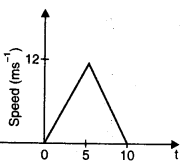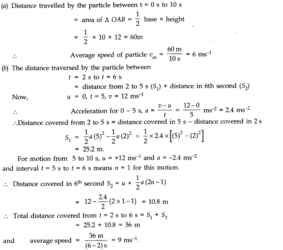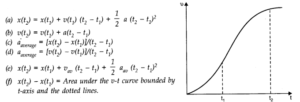# Chapter 3 – Motion in a Straight Line Questions and Answers: NCERT Solutions for Class 11 Physics

Class 11 Physics NCERT book solutions for Chapter 3 - Motion in a Straight Line Questions and Answers.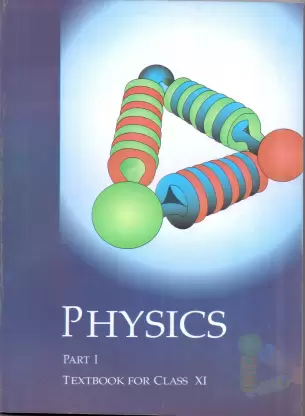## Question 3. 2. The position-time (x -1) graphs for two children A and B returning from their school O to their homes P and Q respectively are shown in Fig. Choose the correct entries in the brackets below: (a) (A/B) lives closer to the school than (B/A). (b) (A/B) starts from the school earlier than (B/A). (c) (A/B) walks faster than (B/A). (d) A and B reach home at the (same/different) time. (e) (A/B) overtakes (B/A) on the road (once/twice).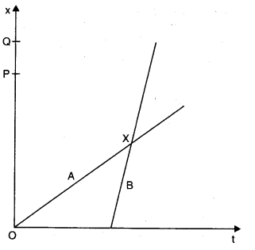## Question 3. 3. A woman starts from her home at 9.00 am, walks with a speed of 5 km h-1on a straight road up to her office 2.5 km away, stays at the office up to 5.00 pm, and returns home by an auto with a speed of 2.5 kmh-1. Choose suitable scales and plot the x-t graph of her motion.

### Answer:Distance covered while walking = 2.5 km. Speed while walking = 5 km/h Time taken to reach office while walking = (2.5/5 ) h=1/2 h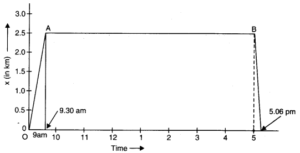## Question 3. 4. A drunkard walking in a narrow lane takes 5 steps forward and 3 steps backward, followed again by 5 steps forward and 3 steps backward, and so on. Each step is 1 m long and requires 1 s. Plot the x-t graph of his motion. Determine graphically and otherwise how long the drunkard takes to fall in a pit 13 m away from the start.

### Answer:Since the man steadily moves forward as the time progresses so the following graph will represent his motion till he covers 13 m. In 5 s he moves through a distance of 5 m and then in next 3 s comes back by 3 m. Thus in 8 s he covers only 2 m, as shown in the graph he would fall in the pit in 37 s.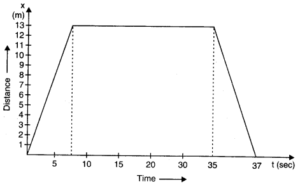## Question 3. 6. A car moving along a straight highway with speed of 126 km h-1is brought to a stop within a distance of 200 m. What is the retardation of the car (assumed uniform), and how long does it take for the car to stop?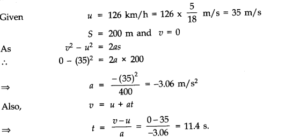## Question 3. 7. Two trains A and B of length 400 m each are moving on two parallel tracks with a uniform speed of 71 km h-1in the same direction, with A ahead of B. The driver of B decides to overtake A and accelerates by 1 ms-1. If after 50 s, the guard of B just brushes past the driver of A, what was the original distance between them?

### Answer:Here length of train A = length of train B = l = 400 m. As speed of both trains u = 72 km h-1= 20 ms-1in same direction, hence their relative velocityuBA= 0. Let initial distance between the two trains be ‘S’ then train B covers the distance (S + 11) = (S + 800) m in time t = 50 s when accelerated with a uniform acceleration a = 1 m/s2.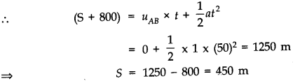## Question 3. 8. On a two-lane road, car A is travelling with a speed of 36 km h-1. Two cars B and C approach car A in opposite directions with a speed of 54 km h-1each. At a certain instant, when the distance AB is equal to AC, both being 1 km, B decides to overtake A before C does. What minimum acceleration of car B is required to avoid an accident?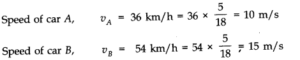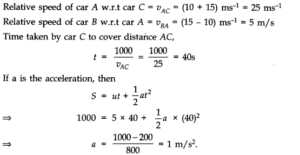## Question 3. 9. Two towns A and B are connected by regular bus service with a bus leaving in either direction every T minute. A man cycling with a speed of 20 km h-1in the direction A to B notices that a bus goes past him every 18 min in the direction of his motion, and every 6 min in the opposite direction. What is the period T of the bus service and with what speed (assumed constant) do the buses ply on the road?

### Answer: Let vb be the speed of each bus. Let vc be the speed of cyclist. Relative velocity of the buses plying in the direction of motion of cyclist is vb – vc . The buses playing in the direction of motion of the cyclist go past him after every 18 minute i.e.18/20 h.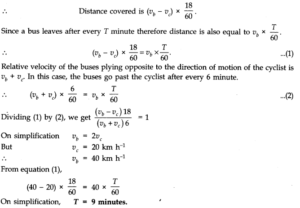## Question 3. 10. A player throws a ball upwards with an initial speed of 29.4 ms-1. (a) What is the direction of acceleration during the upward motion of the ball? (b) What are the velocity and acceleration of the ball at the highest point of its motion? (c) Choose the x = 0 m and t = 0 s to be the location and time of the ball at its highest point, vertically downward direction to be the positive direction of x-axis, and give the signs of position, velocity and acceleration of the ball during its upward, and downward motion. (d) To what height does the ball rise and after how long does the ball return to the player’s hands? (Take g = 9.8 m s-2and neglect air resistance).

### Answer:(a) The direction of acceleration during the upward motion of the ball is vertically downward. (b) At the highest point, velocity of ball is zero but acceleration (g = 9.8 ms-2) in vertically downward direction. (c) If we consider highest point of ball motion as x = 0, t = 0 and vertically downward direction to be +ve direction of x-axis, then (i) during upward motion of ball before reaching the highest point position (as well as displacement) x = +ve, velocity v = -ve and acceleration a = g = +ve. (ii) during the downward motion of ball after reaching the highest point, x, v and a = g all the three quantities are positive. (d) During upward motion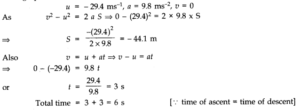## Question 3. 12. A ball is dropped from a height of 90 m on a floor. At each collision with the floor, the ball loses one tenth of its speed. Plot the speed-time graph of its motion between t =0 to 12 s.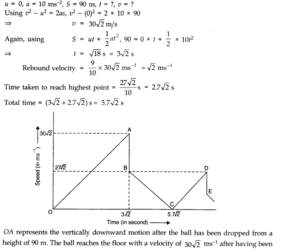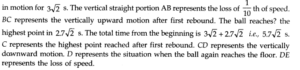## Question 3.14. A man walks on a straight road from his home to a market 2.5 km away with a speed of 5 km h-1.Finding the market closed, he instantly turns and walks back home with a speed of 7.5 km h-1What is the(a)Magnitude of average velocity, and(b)Average speed of the man over the interval of time (i) 0 to 30 min. (ii) 0 to 50 min. (iii) 0 to 40 min? [Note: You will appreciate from this exercise why it is better to define average speed as total path length divided by time, and not as magnitude of average velocity. Youwould not like to tell the tired man on his return home that his average speed was zero!]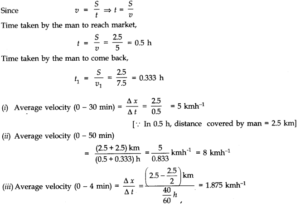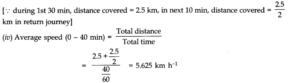## Question 3. 16. Look at the graphs (a) to (d) Fig. carefully and state, with reasons, which of these cannot possibly represent one-dimensional motion of a particle.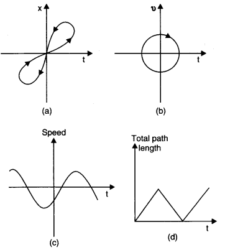## Question 3. 17. Figure shows the x-t plot of one-dimensional motion of a particle. Is it correct to say from the graph that the particle moves in a straight line for t < 0 and on a parabolic path for t > 0? If not, suggest a suitable physical context for this graph.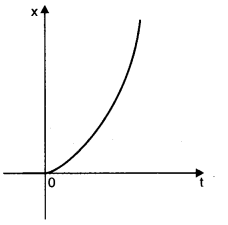## Question 3. 18. A police van moving on a highway with a speed of 30 km h-1fires a bullet at a thief s car speeding away in the same direction with a speed of 192 km h-1. If the muzzle speed of the bullet is 150 ms-1, with what speed does the bullet hit the thief s car? (Note: Obtain that speed which is relevant for damaging the thief s car).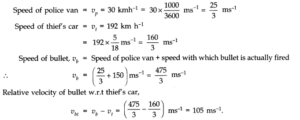## Question 3. 19. Suggest a suitable physical situation for each of the following graphs: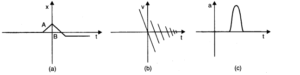## Question 3. 20. Figure gives the x-t plot of a particle executing one¬dimensional simple harmonic motion. (You will learn about this motion in more detail in Chapter 14). Give the signs of position, velocity and acceleration variables of the particle at t = 0.3 s, 1.2 s, – 1.2 s.

### Answer:In x-t graph of Fig. showing simple harmonic motion of a particle, the signs of position, velocity and acceleration are as given below.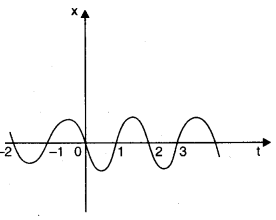## Question 3. 21. Figure gives the x-t plot of a particle in one-dimensional motion. Three different equal intervals of time are shown. In which interval is the average speed greatest, and in which is it the least? Give the sign of average velocity for each interval.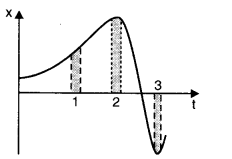## Question 3. 22. Figure gives a speed-time graph of a particle in motion along a constant direction. Three equal intervals of time are shown. In which interval is the average acceleration greatest in magnitude? In which interval is the average speed greatest? Choosing the positive direction as the constant direction of motion, give the signs of v and a in the three intervals. What are the accelerations at points A, B, C and D?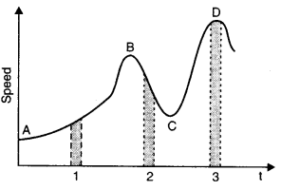## Question 3. 23. A three – wheeler starts from rest , accelerates uniformly with 1 m s-2 on a straight road for 10 s, and then moves with uniform velocity .plot the distance covered by the vehicle during the n th second (n=1,2,3……..) versus n. what do you expect this plot to be during accelerated motion: a straight line or a parabola?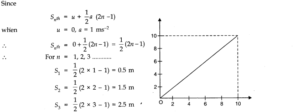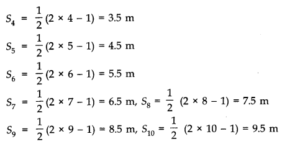## Question 3. 24. A boy standing on a stationary lift (open from above) throws a ball upwards with the maximum initial speed he can, equal to 49 m s-1. How much time does the ball take to return to his hands? If the lift starts moving up with a uniform speed of 5 m s-1and the boy again throws the ball up with the maximum speed he can, how long does the ball take to return to his hands?

### Answer:When either the lift is at rest or the lift is moving either vertically upward or downward with a constant speed, we can apply three simple kinetnatic motion equations presuming a = ± g (as the case may be).In present case u = 49 ms-1(upward) a = g = 9.8 ms-2(downward) If the ball returns to boy’s hands after a time t, then displacement of ball relative to boy is zero i.e., s = 0. Hence, using equation s = ut + 1/2 at 2, we have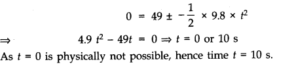## Question 3. 25. On a long horizontally moving belt (Fig.), a child runs to and fro with n speed 9 km h-1(with respect to the belt) between his father and mother located 50 a part on the moving belt. The belt moves with a speed of 4 km h-1. For an observe a stationary platform outside, what is the (a) Speed of the child running in the direction of motion of the belt? (b) Speed of the child running opposite to the direction of motion of the belt? (c) Time taken by the child in (a) and (b)? Which of the answers alter if motion is viewed by one of the parents?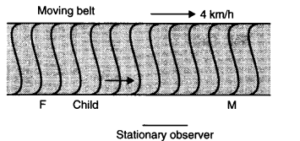## Question 3. 26. Two stones are thrown up simultaneously from the edge of a cliff 200 m high with initial speeds of 15 ms-1and 30 ms-1. Verify that the graph shown in Fig. correctly represents the time variation of the relative position of the second stone with respect to the first. Neglect air resistance and assume that the stones do not rebound after hitting the ground. Take g = 10 ms-2. Give the equations for the linear and curved parts of the plot.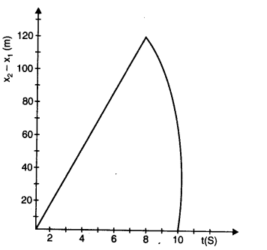## Question 3. 27. The speed-time graph of a particle moving along a fixed direction is shown in Fig. Obtain the distance traversed by the particle between (a) t = 0 s to 10 s. (b) t = 2 s to 6 s. What is the average speed of the particle over the intervals in (a) and (b)?